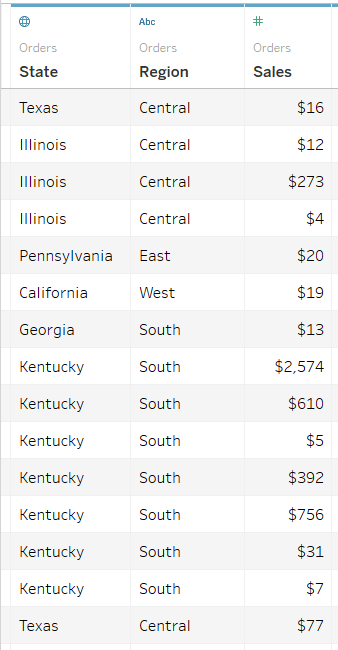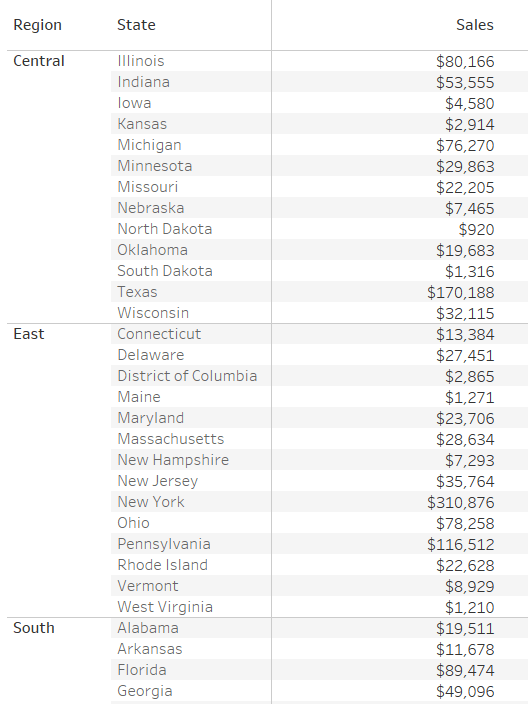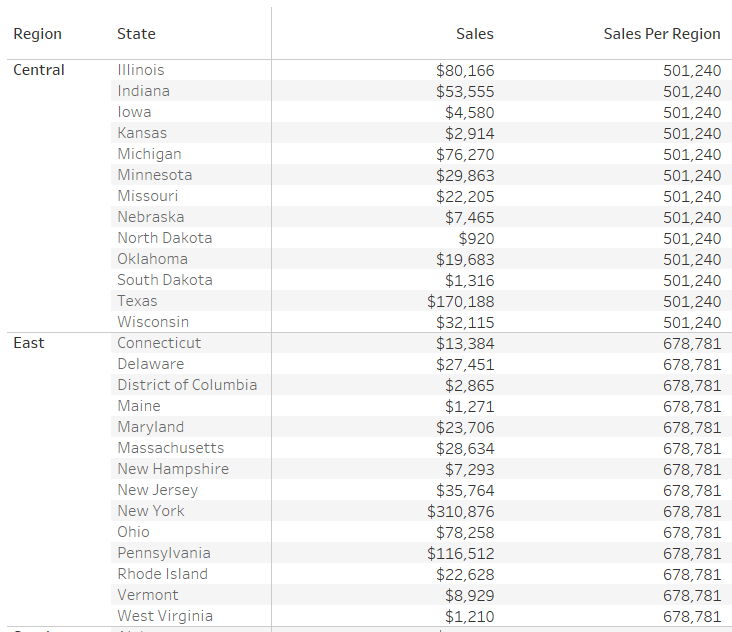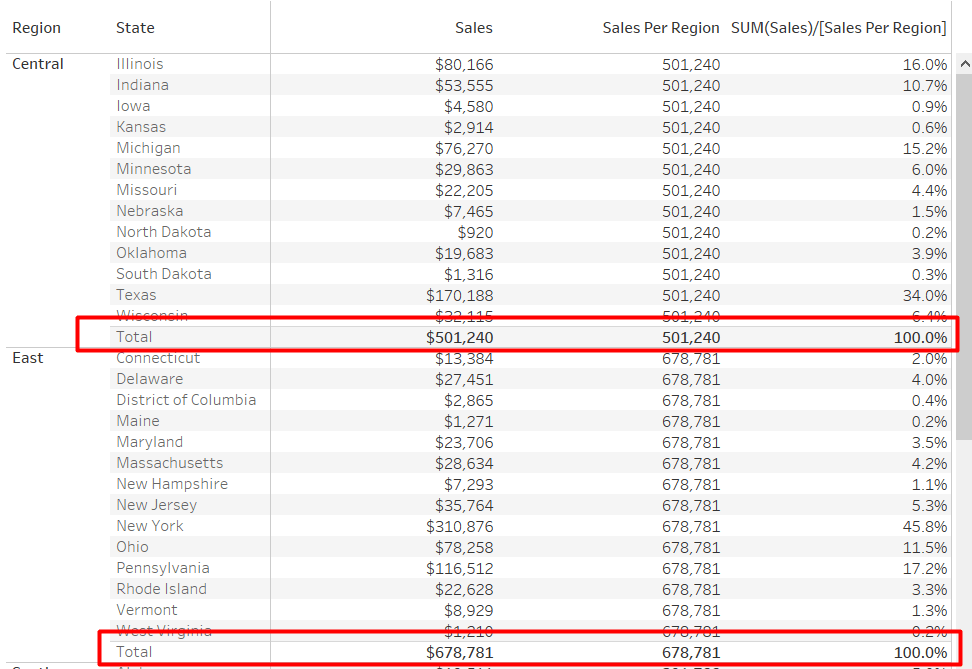# FIXED LOD Calculation – Deep Dive in Tableau

FIXED: “FIXED” level of detail expression aggregates the value only at the dimensions which are specified by the user in the calculation. “FIXED” expression does not take into consideration those dimensions in the view.
The difference between FIXED and INCLUDE/EXCLUDE is unlike INCLUDE/EXCLUDE, FIXED calculations are not relative to the dimensions in view.

Example:
Requirement: Let’s consider the requirement is to calculate the percent distribution of Sales across the different States of Region
Data: The granularity of the data is at “State” level, having other fields like “Region” and “Sales”.Current view: To achieve the required result the first step is to calculate “Sales” across States.But, as the requirement is to show percentage distribution of Sales across States based on Region, we need to use FIXED calculation which can return the total sales across Region, while having States present in the view.
Formula/Expression: SUM({FIXED [Region] : SUM([Sales])})
Explanation:

1. The above calculation will show the sales value across the region. The objective of using FIXED is that even if the user puts another field say “State” in the view, the above calculation still calculates the value at region level only. It will disregard the other dimensions which are in the view i.e. State2. Now, to compare the sales across States we can divide “SUM(Sales)” by “Sales per Region” (which is calculated using the FIXED function)
3. To check the results, we can add “Subtotals” across Regions. As we can see in the below snapshot (as highlighted), the total Sales across all States for that Region is coming out to be same as it is shown in the FIXED calculation of “Sales per Region”:• in
• |
• October 23, 2018#### Author1

Subscribe
Notify of